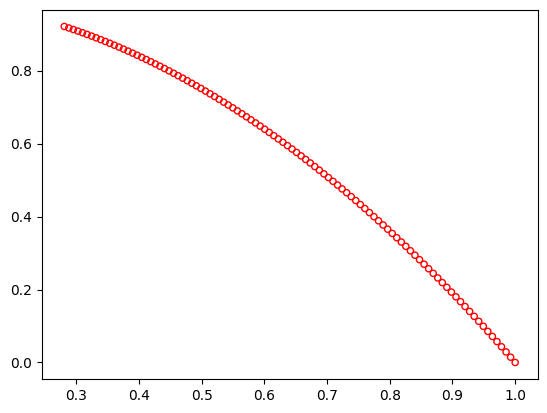# ZDT¶

The ZDT  problem suite is based on the construction process

\begin{align} \begin{split} \min && \; f_1(x)\2mm] \min && \; f_2(x) = g(x) \, h(f_1(x),g(x)) \end{split} \end{align} where two objective have to be minimized. The function $$g(x)$$ can be considered as the function for convergenece and usually $$g(x) = 1$$ holds for pareto-optimal solutions (except for ZDT5). ## ZDT1¶ This is a 30-variable problem ($$n=30$$) with a convex Pareto-optimal set: Definition \begin{align} \begin{split} f_1(x) &= \, & x_1 \\ g(x) &=& 1 + \frac{9}{n-1} \; \sum_{i=2}^{n} x_i \\ h(f_1,g) &=& 1 - \sqrt{f_1/g} \\ \end{split} \end{align} \[0 \leq x_i \leq 1 \quad i=1,\ldots,n

Optimum

$0 \leq x_1^* \leq 1 \quad \text{and} \quad x_i^*=0 \; \text{for} \; i=2,\ldots,n$

Plot

:

from pymoo.factory import get_problem
from pymoo.util.plotting import plot

problem = get_problem("zdt1")
plot(problem.pareto_front(), no_fill=True)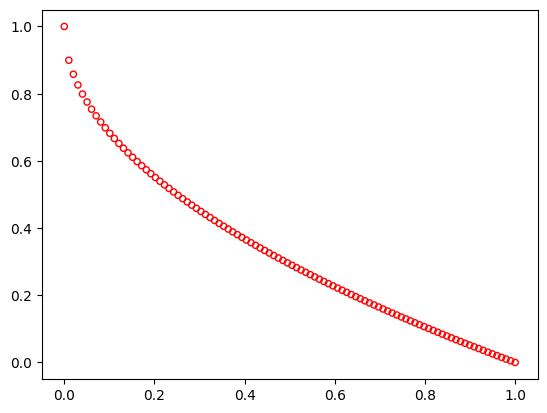## ZDT2¶

This is also a 30-variable problem ($$n=30$$) with a non-convex Pareto-optimal set:

Definition

\begin{align} \begin{split} f_1(x) &= \, & x_1 \\ g(x) &=& 1 + \frac{9}{n-1} \; \sum_{i=2}^{n} x_i \\ h(f_1,g) &=& 1 - (f_1/g)^2 \\ \end{split} \end{align}

$0 \leq x_i \leq 1 \quad i=1,\ldots,n$

Optimum

$0 \leq x_1^* \leq 1 \quad \text{and} \quad x_i^*=0 \; \text{for} \; i=2,\ldots,n$

Plot

:

from pymoo.factory import get_problem
from pymoo.util.plotting import plot

problem = get_problem("zdt2")
plot(problem.pareto_front(), no_fill=True)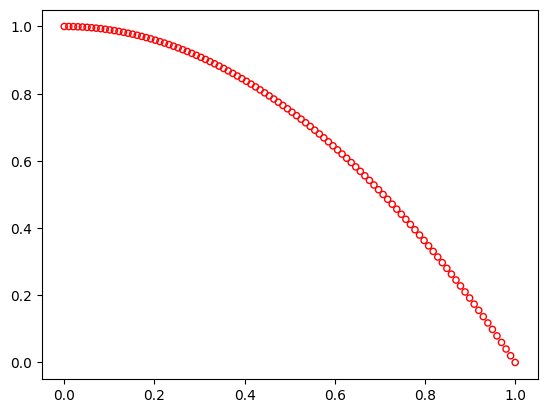## ZDT3¶

This is also a 30-variable problem ($$n=30$$) with a number of disconnected Pareto-optimal fronts:

Definition

\begin{align} \begin{split} f_1(x) &= \, & x_1 \\ g(x) &=& 1 + \frac{9}{n-1} \; \sum_{i=2}^{n} x_i \\ h(f_1,g) &=& 1 - \sqrt{f_1/g} - (f_1/g) \; \sin(10\pi f_1)\\ \end{split} \end{align}

$0 \leq x_i \leq 1 \quad i=1,\ldots,n$

Optimum

$\begin{split}0 \leq x_1^* \leq 0.0830 \\ 0.1822 \leq x_1^* \leq 0.2577\\ 0.4093 \leq x_1^* \leq 0.4538 \\ 0.6183 \leq x_1^* \leq 0.6525 \\ 0.8233 \leq x_1^* \leq 0.8518 \\ x_i^*=0 \; \text{for} \; i=2,\ldots,n\end{split}$

Plot

:

from pymoo.factory import get_problem
from pymoo.util.plotting import plot

problem = get_problem("zdt3")
plot(problem.pareto_front(), no_fill=True)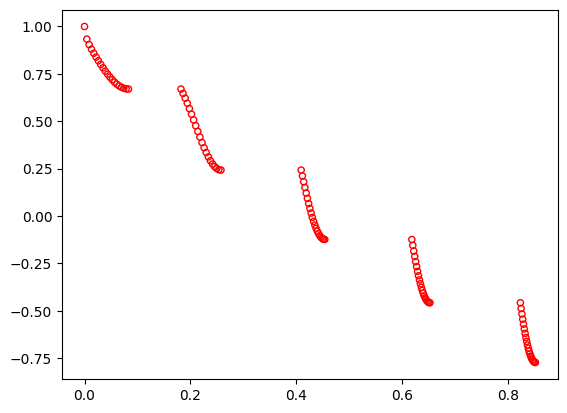## ZDT4¶

This is a 10-variable ($$n=10$$) problem having a convex Pareto-optimal set. There exist many local Pareto-optimal solutions in this problem. Therefore, algorithms can easily get stuck in a local optimum.

Definition

\begin{align} \begin{split} f_1(x) &= \, & x_1 \\ g(x) &=& 1 + 10(n-1) + \sum_{i=2}^{n} (x_i^2 - 10 \cos(4\pi x_i))\\ h(f_1,g) &=& 1 - \sqrt{f_1/g}\\ \end{split} \end{align}

$\begin{split}0 \leq x_1 \leq 1 \\ -10 \leq x_i \leq 10 \quad i=2,\ldots,n\end{split}$

Optimum

$0 \leq x_1^* \leq 1 \quad \text{and} \quad x_i^*=0 \; \text{for} \; i=2,\ldots,n$

Plot

:

from pymoo.factory import get_problem
from pymoo.util.plotting import plot

problem = get_problem("zdt4")
plot(problem.pareto_front(), no_fill=True)## ZDT5¶

In ZDT5 in variables are decodec by bitsrings. At all 11 discrete variables are used, where $$x_1$$ is represented by 30 bits and the rest $$x_2$$ to $$x_{11}$$ by 5 bits each. The function $$u(x)$$ does nothing else than count the number of $$1$$ of the corresponding variable. Also, note that the objective function is deceptive, because the values of $$v(u(x_i))$$ are decreasing with the number of 1’s, but has its minimum when all variables are indeed 1.

Definition

\begin{align} \begin{split} f_1(x) &= \, & 1+u(x_1)\\ g(x) &=& \sum_{i=2}^{n} v(u(x_i))\2mm] v(u(x_i)) &=& \begin{cases} 2 + u(x_i) \quad \text{if} \; u(x_i) < 5\\ 1 \quad \quad \quad \; \; \; \text{if} \; u(x_i) = 5 \end{cases}\\[4mm] h(f_1,g) &=& 1 /f_1(x)\\[2mm] \end{split} \end{align} Optimum \[0 \leq u(x_1^*) \leq 30 \quad \text{and} \quad u(x_i^*)=5 \; \text{for} \; i=2,\ldots,n

Plot

:

from pymoo.factory import get_problem
from pymoo.util.plotting import plot

problem = get_problem("zdt5", normalize=False)
plot(problem.pareto_front(), no_fill=True)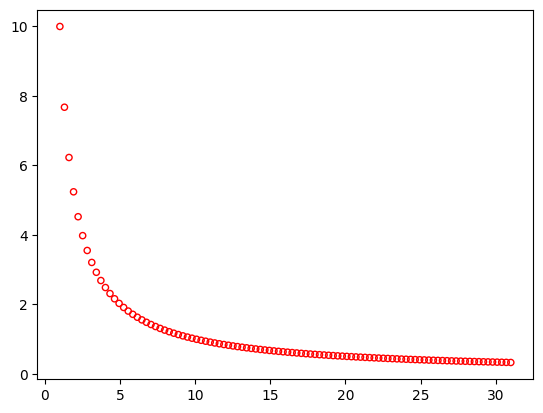Please note that by default here the Pareto-front is not normalized. However, this can be easily achived normalizing $$f_1$$ in the range of $$(1,31)$$ and $$f_2$$ in $$(10/31, 10)$$ which are the known bounds of the Pareto-set. By default the normalized problem is used.

:

from pymoo.factory import get_problem
from pymoo.util.plotting import plot

problem = get_problem("zdt5", normalize=False)
plot(problem.pareto_front(), no_fill=True)## ZDT6¶

This is a 10-variable ($$n=10$$) problem having a nonconvex Pareto-optimal set. The density of solutions across the Pareto-optimal region is non-uniform.

Definition

\begin{align} \begin{split} f_1(x) &= \, & 1 - \exp(-4 x_1) \sin^6 (6 \pi x_1) \2mm] g(x) &=& 1 + 9 \bigg[\bigg( \sum_{i=2}^{n} x_i \bigg) / 9\bigg]^{0.25}\\[2mm] h(f_1,g) &=& 1 - (f_1/g)^2\\ \end{split} \end{align} \[0 \leq x_i \leq 1 \quad i=1,\ldots,n

Optimum

$0 \leq x_1^* \leq 1 \quad \text{and} \quad x_i^*=0 \; \text{for} \; i=2,\ldots,n$

Plot

:

from pymoo.factory import get_problem
from pymoo.util.plotting import plot

problem = get_problem("zdt6")
plot(problem.pareto_front(), no_fill=True)